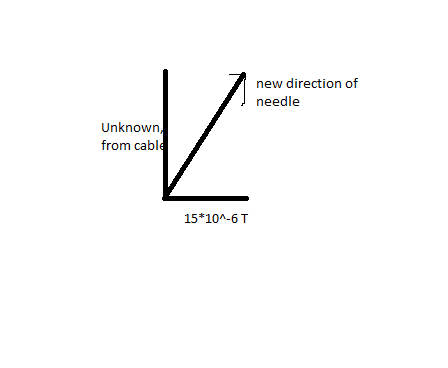# Compass needle, magnetic field and current

## Homework Statement

Eric looks at his compass to check the direction to north. At a point two (2.0) meters below Eric, a cable is drawn from south to north and the current running through the cable is 50 Amperes. This current makes the compass needle turn wrong. How big is the angle deviation if the horizontal component of earth's magnetic field has the value 15*10^-6 Tesla?

## Homework Equations

We know the distance between the compass and the cable, since I don't know which formula that can be applied yet I will just call it a = 2.0 meters.
Current, I = 50 A. Horizontal component of magnetic field from earth, 15*10^-6 Tesla.

Some equations that could help:
B = k * I/a where k is a constant 2.0*10^-7 Tm/A.

## The Attempt at a Solution

He is standing directly above the cable which points to north. We know the horizontal component of the magnetic field from earth, but wouldn't that mean the compass needle points to the right or left (since it is a horizontal component) and therefore he isn't facing north?

Let's forget what I just said and still try do attempt at some sort of solution.Using the formula we have, we'd get that B = k * I/a. So =>
2.0*10^-7 * (50/2) = 2.0*10^-7 * 25 = 5*10^-6. So the vertical component is that.

Now to calculate the angle between the vertical component and the resulting vector ("hypothenuse") we use tan^-1 ((15*10^-6)/(5*10^-6)) and I get 71.57 degrees. So the total difference caused by the cable is 71.57 degrees.

I strongly doubt this, since a lot had to be assumed. Where did I go wrong?

mfb
Mentor
So the vertical component is that.
What do you mean with "vertical"?
So the total difference caused by the cable is 71.57 degrees.
Be careful what the 72 degrees are relative to.
Simple cross-check: 15 is larger than 5, so is the resulting field closer to the direction of the magnetic field of earth or the one from the cable?
Apart from that, it looks good.

What do you mean with "vertical"?
Be careful what the 72 degrees are relative to.
Simple cross-check: 15 is larger than 5, so is the resulting field closer to the direction of the magnetic field of earth or the one from the cable?
Apart from that, it looks good.

With vertical I mean the component that goes with the cable. I don't really know how the compass needle moves, I can only assume it deviates 72 degrees from whatever point it started, but I don't know if it is to the right or the left. How do I think here?

mfb
Mentor
With vertical I mean the component that goes with the cable.
Is it really vertical in the 3D world? Like, going upwards/downwards?

I can only assume it deviates 72 degrees from whatever point it started
Why?
You have a (relatively) large magnetic field from earth, and a small deviation from the cable. Just draw them on paper and see if 72° are realistic. Also, check the arguments of your tan to see if they agree with that sketch.

but I don't know if it is to the right or the left
That is fine, the current direction is unknown anyway.

Is it really vertical in the 3D world? Like, going upwards/downwards?

Why?
You have a (relatively) large magnetic field from earth, and a small deviation from the cable. Just draw them on paper and see if 72° are realistic. Also, check the arguments of your tan to see if they agree with that sketch.

That is fine, the current direction is unknown anyway.
Now that I've checked solutions, it appears that the component that goes to the "right" is from the cable and earth's magnet field upwards, even though the problem states the magnetic field's effect is horizontal. Why?

mfb
Mentor
Why do you think any (relevant) component goes upwards? For the magnetic field of earth, the problem statement explicitely says "horizontal".

Why do you think any (relevant) component goes upwards? For the magnetic field of earth, the problem statement explicitely says "horizontal".
We have been taught to think that way. Is there any other way?

mfb
Mentor
We have been taught to think that way.
Where, how?
Any other way compared to what?

rude man
Homework Helper
Gold Member
Now that I've checked solutions, it appears that the component that goes to the "right" is from the cable and earth's magnet field upwards, even though the problem states the magnetic field's effect is horizontal. Why?
The earth's magnetic field is mostly horizontal near the equator and mostly vertical near the poles. But your problem asks you to forget the vertical component so if it makes you happy just pretend you're at the equator ...